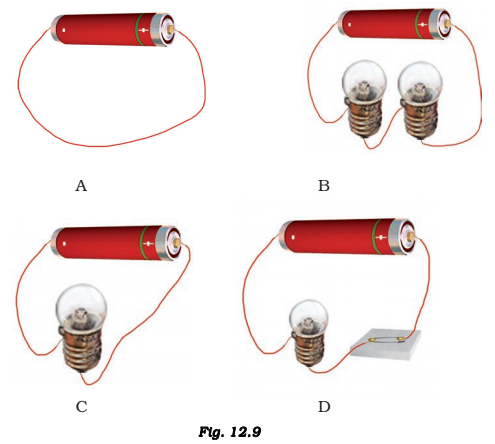Which of the following arrangement A, B, C and D given in fig 12.9 should not be set up? Explain, why?(A) is the inappropriate setup.

• In figure B the electric cell is connected to two bulbs and both bulbs will consume the cell at a very high rate but we are getting more light if we connect two bulbs.
• In figure C the cell is connected to a bulb which will consume less electricity than the two bulbs and it will also give us light.
• In figure D the cell is connected to a bulb using a switch which is the most appropriate way to connect the bulb, it will use the electric cell at very low rate.(2)(2)